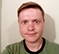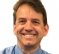# A New Contender in the Digital Differentiator Race

This blog proposes a novel differentiator worth your consideration. Although simple, the differentiator provides a fairly wide 'frequency range of linear operation' and can be implemented, if need be, without performing numerical multiplications.

## Background

In reference  I presented a computationally-efficient tapped-delay line digital differentiator whose $h_{ref}(k)$ impulse response is:

$$h_{ref}(k) = {-1/16}, \ 0, \ 1, \ 0, \ {-1}, \ 0, \ 1/16 \tag{1}$$

and whose $\lvert H_{ref}(\omega) \rvert$ frequency magnitude response is shown by the blue dashed curve in Figure 1.Figure 1: Normalized frequency magnitude responses of
the reference  differentiator, and a
central-difference differentiator.

For comparison purposes, Figure 1 shows the frequency magnitude response of the popular central-difference differentiator (green dotted curve), $\lvert H_{cd}(\omega) \rvert$, whose $h_{cd}(k)$ impulse response is:

$$h_{cd}(k) = 1/2, \ 0, \ {-1/2}. \tag{2}$$

In Figure 1 we see the $\lvert H_{ref}(\omega) \rvert$ response's increased 'frequency range of linear operation' compared to the $\lvert H_{cd}(\omega) \rvert$ frequency magnitude response. That's the primary benefit of the $h_{ref}(k)$ differentiator.

The response curves in Figure 1 are what I call "normalized" magnitude responses. I'll define what I mean by normalized later in this blog.

(As a bit of trivia, it's interesting to note that the central-difference differentiator's magnitude response is equal to the first half cycle of a sine wave. I prove that fact in Appendix A.)

## The Proposed Differentiator

The improved differentiator I propose here has the impulse response given by:

$$h_{pro}(k) = {-3/16}, \ 31/32, 0, \ {-31/32}, \ 3/16 \tag{3}$$

and whose $\lvert H_{pro}(\omega) \rvert$ frequency magnitude response is shown by the black solid curve in Figure 2.Figure 2: Normalized frequency magnitude responses of
the proposed differentiator, the reference 
differentiator, and a central-difference
differentiator.

Figure 2 shows the benefit of the proposed $h_{pro}(k)$ differentiator. Its $\lvert H_{pro}(\omega) \rvert$ response has an increased-width (by roughly 33%) 'frequency range of linear operation' compared to the $\lvert H_{ref}(\omega) \rvert$ frequency magnitude response. Based on Figure 2, the $h_{pro}(k)$ differentiator should be useful in applications where the spectral components of the input signal are less than $\pi/2$ radians/sample $(f_s/4 \ Hz.)$

The $h_{pro}(k)$ differentiator has, due to its antisymmetrical coefficients, linear phase in the frequency domain. In addition, the differentiator's input/output time delay (group delay) is exactly two sample periods. Having a delay that's an integer number of samples makes this differentiator useful when its output must be synchronized with other time-domain sequences, such as in FM demodulation applications.

## Proposed Differentiator Implementation

The traditional tapped-delay line implementation of the proposed differentiator is shown in Figure 3(a). A folded delay line implementation reducing the number of computations from four multiplications to two multiplications per output sample is given in Figure 3(b). And a folded multiplier-free implementation is shown in Figure 3(b) where multipliers are replaced by binary right-shift operations. In that figure the 'BRS,x' nomenclature means a binary right shift by x bits.Figure 3: Proposed $h_{pro}(k)$ differentiator implementations:
implementation; (c) folded multiplier-free
implementation.

## Differentiator Gains and Normalized Magnitude Curves

For completeness I mention that the $h_{ref}(k)$ and $h_{pro}(k)$ differentiators have magnitude response gains greater than unity. This is shown by the actual frequency magnitude responses shown in Figure 4.

To explain what I mean by "gain", notice how the slope of the central-difference differentiator's $\lvert H_{cd}(\omega) \rvert$ response (green dotted) curve is unity at low frequencies in Figure 4. Thus I claim the gain of the $h_{cd}(k)$ differentiator has a gain of one. The slope of the reference  differentiator's $\lvert H_{ref}(\omega) \rvert$ response (blue dashed) curve is 1.63 at low frequencies and the slope of the proposed differentiator's $\lvert H_{pro}(\omega) \rvert$ response (black solid) curve is 1.2 at low frequencies. So I claim that the $h_{ref}(k)$ reference  and $h_{pro}(k)$ proposed differentiators have gains of 1.63 and 1.2 respectively.Figure 4: Actual frequency magnitude responses of
the proposed differentiator, the reference 
differentiator, and a central-difference
differentiator.

Those actual magnitude response curve in Figure 4 prevent us from comparing the 'frequency ranges of linear operation' of the various differentiators. So to make that comparison I divided the Figure 4 $\lvert H_{ref}(\omega) \rvert$ response sample values by 1.63 to create the normalized blue dashed curves in Figures 1 and 2. Likewise, I divided the Figure 4 $\lvert H_{pro}(\omega) \rvert$ response sample values by 1.2 to create the normalized solid black curve in Figure 2.

## A Digital Differentiator Clarification

When thinking about digital differentiators it's useful to be aware of the algebraic form of their time-domain difference equations. For example, let's assume that $y(n)$ represents a central-difference derivative of an $x(n)$ sequence. The correct estimation of the $y(n)$ derivative of an $x(n)$ is:

$$\text{estimated derivative of } x(n) = y(n) = {{x(n) - x(n-2)} \over {2t_s}} \tag{4}$$

where $t_s$ is the time between the $x(n)$ samples measured in seconds. (Variable $t_s$ is the 'dt' in a generic differentiator's dx/dt derivative.)

Often in the DSP literature, for convenience, the time between $x(n)$ samples is assumed to be unity, i.e. $t_s = 1$ and a central-difference differentiator's difference equation takes the popular form of:

$$y'(n) = {{x(n) - x(n-2)} \over 2} . \tag {5}$$

Computing Eq. (5)'s estimated $y'(n)$ derivative is acceptable in many applications because $y'(n)$ will always be proportional to Eq. (4)'s $y(n)$. But to be dimensionally correct, Eq. (4)'s $y(n)$ should be computed, rather than Eq. (5)'s $y'(n)$.

For example, let's say the continuous red-dotted curve in Figure 5 is the $x(t)$ instantaneous water pressure inside a water pipe measured in pounds per square inch (psi). And we have three $x(n)$ samples of $x(t)$ shown by the large $x(n)$ black dots. The $t_s$ time between sample is 0.5 seconds.Figure 5: Digital differentiator example. Estimating the
slope, $dx(t)/dt$, of the continuous $x(t)$ signal
by way of digital differentiation of the
sampled $x(n)$ sequence.

To estimate the rate of pressure change at time instant two seconds $(4t_s)$ once sample $x(5)$ is available, using Eq. (5) gives us a $y'(5)$ value of:

$$y'(5) = {{x(5)-x(3)} \over 2} = {{10 \ psi - 5 \ psi} \over 2} = 2.5 psi.$$

However, time rate of pressure change values must dimensionally be psi/second.

What we should do is use Eq. (4) giving us a dimensionally-correct time rate of pressure change value of:

$$y(5) = {{x(5) - x(3)} \over {2t_s \ seconds}} = {{10 \ psi - 5 \ psi} \over {1 \ second}} = 5 \ psi/second.$$

Result $y(5)$ tells us the water pressure increased by 5 psi during the one-second time interval from 1.5 seconds to 2.5 seconds. So my point is, Eq. (5)'s $y'(n)$ is the popular form of a central-difference differentiator's difference equation, but Eq. (4)'s $y(n)$ expression is the dimensionally-correct form.## Appendix A: Proof of Cent-Diff Differentiator's Sinusoidal Magnitude Response

The proof that a central-difference differentiator's magnitude response is equal to the magnitude of a sine wave proceeds as follows: With the differentiator's time-domain impulse response being $h_{cd}(k) = [1/2, \ 0, \ -1/2]$, its z-transform is

$$H_{cd}(z) = {{z^{-0} - z^{-2}} \over 2} . \tag{A-1}$$

Multiplying Eq. (A-1) by $z/z$ gives us a new, and equivalent, z-transform of:

$$H_{cd}(z) = {1 \over z}{{z^{1} - z^{-1}} \over 2} . \tag{A-2}$$

Replacing $z$ with $e^{j\omega}$ gives us the differentiator's frequency response of:

$$H_{cd}(\omega) = {1 \over {e^{j\omega}}}{{e^{j\omega} - e^{-j\omega}} \over 2} . \tag{A-3}$$

Knowing that $(e^{-j\omega} -e^{-j\omega})/2 = jsin(\omega)$, we write:

$$H_{cd}(\omega) = {j \over {e^{j\omega}}}[sin(\omega)] = {{e^{j\pi/2}} \over {e^{j\omega}}}[sin(\omega)] . \tag{A-4}$$

Therefore the $\lvert H_{cd}(\omega) \rvert$ frequency magnitude response is equal to the magnitude of a sine wave as:

$$\lvert H_{cd}(\omega) \rvert = \left\lvert\frac{e^{j\pi/2}}{e^{j\omega}}[sin(\omega)]\right\rvert = \left\lvert {e^{-j(\omega-\pi/2)}[sin(\omega)]} \right\rvert = \lvert sin(\omega) \rvert . \tag{A-5}$$

[ - ]
Comment by June 28, 2016Hello mhilgenforf,
perhaps you didn't take into account the 'normalization' that I described in my blog. Try the following MATLAB code and if you're still having problems please let me know.
[-Rick-]

clear
h_CentDiff = [1, 0, -1]/2;
h_Prop = [-3/16, 31/32, 0, -31/32, 3/16];
h_Prop_Norm = 0.7855*[-3/16, 31/32, 0, -31/32, 3/16];

[Freq_Resp_CentDiff,Freq] = freqz(h_CentDiff,1);
[Freq_Resp_Prop,Freq] = freqz(h_Prop,1);
[Freq_Resp_Prop_Norm,Freq] = freqz(h_Prop_Norm,1);

Mag_CentDiff = abs(Freq_Resp_CentDiff);
Mag_Prop = abs(Freq_Resp_Prop);
Mag_Prop_Norm = abs(Freq_Resp_Prop_Norm);

figure(1), clf
subplot(2,1,1)
plot([0, pi],[0, pi],':k') % Ideal freq mag response
hold on
plot(Freq, Mag_CentDiff,'g', Freq, Mag_Prop,'k')
hold off
axis([0, pi, 0, 3])
ylabel('Gain'), grid on, zoom on
title('CenDiff = green, Proposed = black')

subplot(2,1,2)
plot([0, pi],[0, pi],':k') % Ideal freq mag response
hold on
plot(Freq, Mag_CentDiff,'g', Freq, Mag_Prop_Norm,'k')
hold off
axis([0, pi, 0, 3]), xlabel('Freq (Radians/Sample)')
ylabel('Gain'), grid on, zoom on
title('CenDiff = green, Proposed-Normalized = black')
[ - ]
Comment by June 27, 2016Excuse my ignorance, but if you take the coefficients you give in the signal flow diagram (a) and plug them into MATLAB the computed frequency response is very different from that shown in Fig. 2.
[ - ]
Comment by June 15, 2022Nice article Rick!

I see that Neil also wrote an article summarizing various differentiators and quantifying their noise performance; I was curious if such an article (or perhaps this is in one of your books) exists summarizing the mean error for both magnitude and phase for different discrete time differentiators?

Phase is often overlooked but can be an important consideration; I was particularly curious how a Tustin (Bilinear Transform) mapped differentiator would compare in the pile of options since that would provide an exact phase match over all frequencies (and distorts the amplitude due to the "frequency compression" property of that mapping technique). A Tustin mapped differentiator would be implemented as:

H(s) = s

H(z) = (z-1)/(z+1)

y[n] = x[n] - x[n-1] - y[n-1]

[ - ]
Comment by June 19, 2022